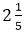# RD Sharma Class 6 ex 6.9 Solutions Chapter 6 Fractions

In this chapter, we provide RD Sharma Class 6 ex 6.9 Solutions Chapter 6 Fractions for English medium students, Which will very helpful for every student in their exams. Students can download the latest RD Sharma Class 6 ex 6.9 Solutions Chapter 6 Fractions Maths pdf, Now you will get step by step solution to each question.

# Chapter 6: Fractions – Exercise 6.9

### Question: 1

i) 3/4 and 5/6

ii) 7/10 and 2/15

iii) 8/13 and 2/3

iv) 4/5  and  7/15

### Solution:

i) Given: 3/4 and 5/6

LCM of 4 and 6 is 12, so we will convert each fraction into an equivalent fraction with denominator 12.

ii) Given: 7/10 and 2/15

LCM of 10 and 15 is 30, so we will convert each fraction into an equivalent fraction with denominator 30.

iii) Given: 8/13 and 2/3

LCM of 13 and 3 is 39, so we will convert each fraction into an equivalent fraction with denominator 39.

iv) Given: 4/5 and 7/15

LCM of 5 and 15 is 1, so we will convert each fraction into an equivalent fraction with denominator 15.

Subtract:

### Solution:

i) Given: 2/7 and  19/21

LCM of 21 and 7 is 21, so we will convert each fraction into an equivalent fraction with denominator 21.

ii) Given: 21/25 and 18/20

LCM of 20 and 25 is 100, so we will convert each fraction into an equivalent fraction with denominator 100.

iii) Given: 2/1 and 7/16

LCM of 1 and 16 is 16, so we will convert each fraction into an equivalent fraction with denominator 16.

LCM of 5 and 15 is 15, so we will convert each fraction into an equivalent fraction with denominator 15.

### Question: 3

Find the difference of:

i) 13/24 and 7/16

i)  5/18 and 4/15

i)  1/12 and 3/4

i) 2/3 and 6/7

### Solution:

i) Given: 13/24 and 7/16

ii) Given: 5/18 and 4/15

iii) Given: 3/4 and 1/12

iv) Given: 6/7 and 2/3

### Question: 4

Subtract as indicated:

### Solution:

i) Given: 8/3 and 5/9

Simplify:

### Question: 6

Replace * with a correct number:

Therefore, 7/8

### Question: 7

Savita bought 2/5 m of ribbon and kavita 3/4 m of ribbon. What was the total length of the ribbon they bought?

### Solution:

Length of the ribbon bought by savita = 2/5 metres

Length of the ribbon bought by kavita = 3/4 metres

Total length of the ribbon bought by them:

### Question: 8

Ravish takesminutes to walk across the school ground. Rahul takes 7/4 minutes to do the same. Who takes less time and by what fraction?

### Solution:

(LCM of 4 and 5 is 20, so will we convert each fraction into an equivalent fraction with denominator 20)

Rahul takes less time,

### Question: 9

A piece of a wire 7/8 metres long broke into two pieces. One piece was 1/4 meter long. How long is the other piece?

### Solution:

Length of the wire = 7/8 metres

Length of one piece of wire = 1/4 metres

Let the length of the second piece of wire be x m.

Therefore, Length of the wire = length of one piece + length of the second piece

Therefore, the length of the second piece is 5/8 metres

### Question: 10

Shikha and priya have bookshelves of the same size shikha’s shelf is 5/6 full of book and priya’s shelf is 2/5 full. Whose bookshelf is more full? By what fraction?

### Solution:

Fraction of shikha’s filled bookshelf = 5/6

Fraction of Priya’s filled bookshelf = 2/5

Comparing 5/6  and 2/5, we get :

LCM of 5 and 6 is 30, so will convert each fraction into an equivalent fraction with denominator 30.

Shikha’s shelf is more full.

Therefore,

### Question: 11

Ravish’s house is 9/10 Km from his school. He walked some distance and then took a bus for 1/2 Km. How far did he walk?

### Solution:

Total distance between the house and the school = 9/10 Km

Distance covered in the bus = 1/2 Km

Distance covered by walking + distance covered in the bus = total distance between

the house and the school

Distance covered by walking = total distance between the house and the school – Distance

covered in the bus

Distance covered by walking:

9/10 Km – 1/2 Km

LCM of 10 and 2 is 10, so we convert each fraction into an equivalent fraction with denominator 10

(HCF of numerator and denominator is 2)

All Chapter RD Sharma Solutions For Class 6 Maths

I think you got complete solutions for this chapter. If You have any queries regarding this chapter, please comment on the below section our subject teacher will answer you. We tried our best to give complete solutions so you got good